## Resampling, the jackknife, and pseudo-observations

Resampling methods approximate the sampling distribution of a statistic or estimator. In essence, a sample taken from the population is treated as a population itself. A large number of new samples, or resamples, are taken from this “new population”, commonly with replacement, and within each of these resamples, the estimate of interest is re-obtained. A large number of these estimate replicates can then be used to construct the empirical sampling distribution from which confidence intervals, bias, and variance may be estimated. These methods are particularly advantageous for statistics or estimators for which no standard methods apply or are difficult to derive.

The jackknife is a popular resampling method, first introduced by Quenouille in 1949 as a method of bias estimation. In 1958, jackknifing was both named by Tukey and expanded to include variance estimation. A jackknife is a multipurpose tool, similar to a swiss army knife, that can get its user out of tricky situations. Efron later developed the arguably most popular resampling method, the bootstrap, in 1979 after being inspired by the jackknife.

Good simple ideas, of which the jackknife is a prime example, are our most precious intellectual commodity, so there is no need to apologize for the easy mathematical level.

Despite existing since the 1940’s, resampling methods were infeasible due to the computational power required to perform resampling and recalculate estimates many times. With today’s computing power, the uncomplicated yet powerful jackknife, and resampling methods more generally, should be a tool in every analyst’s toolbox.

## Simplifying U-statistic variance estimation with Sen’s structural components

Sen (1960) proved that U-statistics could be decomposed into identically distributed and asymptotically uncorrelated “structural components.”

The mean of these structural components is equivalent to the U-statistic and the variance of the structural components can be used to estimate the variance of the U-statistic, bypassing the need for often challenging derivation of conditional variance components.

## One, Two, U: Examples of common one- and two-sample U-statistics

My previous two blog posts revolved around derivation of the limiting distribution of U-statistics for one sample and multiple independent samples.

For derivation of the limiting distribution of a U-statistic for a single sample, check out Getting to know U: the asymptotic distribution of a single U-statistic.

For derivation of the limiting distribution of a U-statistic for multiple independent samples, check out Much Two U About Nothing: Extension of U-statistics to multiple independent samples.

The notation within these derivations can get quite complicated and it may be a bit unclear as to how to actually derive components of the limiting distribution.In this blog post, I provide two examples of both common one-sample U-statistics (Variance, Kendall’s Tau) and two-sample U-statistics (Difference of two means, Wilcoxon Mann-Whitney rank-sum statistic) and derive their limiting distribution using our previously developed theory.

## Much Two U About Nothing: Extension of U-statistics to multiple independent samples

Thank you very much to the lovely Feben Alemu for pointing me in the direction of https://pungenerator.org/ as a means of ensuring we never have to go without a brilliant title! With great power comes great responsibility.## Review

Statistical functionals are any real-valued function of a distribution function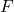,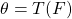. Whenis unknown, nonparametric estimation only requires thatbelong to a broad class of distribution functions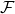, typically subject only to mild restrictions such as continuity or existence of specific moments.

For a single independent and identically distributed random sample of size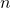,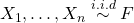, a statistical functionalis said to belong to the family of expectation functionals if:

1.takes the form of an expectation of a function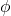with respect to,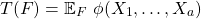2.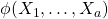is a symmetric kernel of degree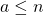.

A kernel is symmetric if its arguments can be permuted without changing its value. For example, if the degree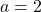,is symmetric if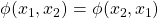.

Ifis an expecation functional and the class of distribution functionsis broad enough, an unbiased estimator ofcan always be constructed. This estimator is known as a U-statistic and takes the form,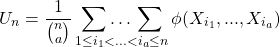such that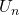is the average ofevaluated at all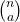distinct combinations of size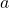from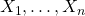.

For more detail on expectation functionals and their estimators, check out my blog post U-, V-, and Dupree statistics.

Since each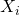appears in more than one summand of, the central limit theorem cannot be used to derive the limiting distribution ofas it is the sum of dependent terms. However, clever conditioning arguments can be used to show thatis in fact asymptotically normal with mean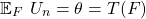and variance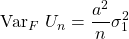where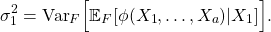The sketch of the proof is as follows:

1. Express the variance ofin terms of the covariance of its summands,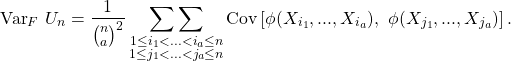1. Recognize that if two terms share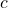common elements such that,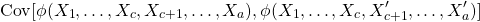conditioning on theirshared elements will make the two terms independent.

2. For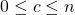, define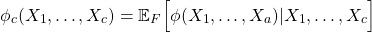such that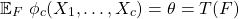and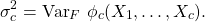Note that when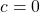,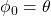and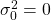, and when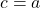,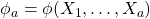and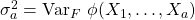.

3. Use the law of iterated expecation to demonstrate that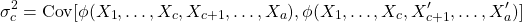and re-express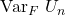as the sum of the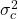,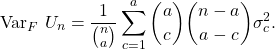Recognizing that the first variance term dominates for large, approximate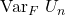as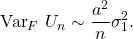4. Identify a surrogate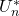that has the same mean and variance as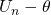but is the sum of independent terms,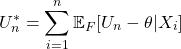so that the central limit may be used to show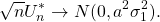5. Demonstrate that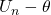and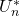converge in probability,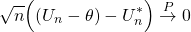and thus have the same limiting distribution so that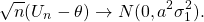For a walkthrough derivation of the limiting distribution offor a single sample, check out my blog post Getting to know U: the asymptotic distribution of a single U-statistic.

This blog post aims to provide an overview of the extension of kernels, expectation functionals, and the definition and distribution of U-statistics to multiple independent samples, with particular focus on the common two-sample scenario.